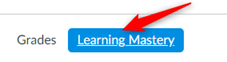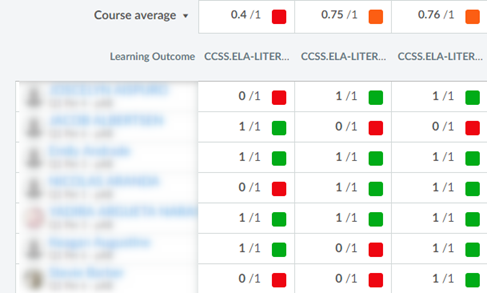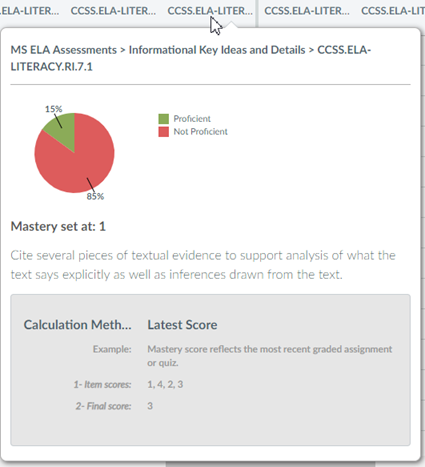Click Settings from the Course Navigation (bottom left)From the Feature Options tab, toggle the Learning Mastery Gradebook button onThe Learning Mastery Gradebook is organized like the Assignments Gradebook with the student names on the left, but each column consists of a course outcome and the outcome grade for each student along with the course average or median above each outcome.

Individual student scores within each outcome are based on outcome values. The first number indicates the score the student earned. The second number indicates the mastery threshold, which is the minimum the students need to achieve mastery for the outcome. For instance, if a student earns a score of 5/3, the student has earned 2 points above the base mastery threshold of 3 points. If a student achieves a score of 1/3, the student has not achieved enough points to reach the mastery threshold.For Middle School Literacy Assessments, each outcome’s mastery level has been set to 1.  Almost all outcomes are linked to only 1 question.  However, a few outcomes are linked to 2 questions.

The first two columns (outcomes) are linked to only 1 question.  The third column (outcome) is linked to two questions.

I will explain how to read the scores below for the 3 students show above.

First student:

• Answered all questions correct as their score was 1/1 for each outcome.

Second student:

• Answered the question for the 1st outcome listed incorrect as the score was 0/1
• Answered the question for the 2nd outcome listed correct as the score was 1/1
• Answered both questions for the 3rd outcome listed incorrect as the score was 0/1

Third student:

• Answered the question for the 1st outcome listed correct as the score was 1/1
• Answered the question for the 2nd outcome listed correct as the score was 1/1
• Answered one question correct and one question incorrect for the 3rd outcome listed as the score was 0.5/1Hover over the outcome title to view a breakdown of a specific outcome. The circle graph shows how the individual student scores were divided into the outcomes criterion ratings.